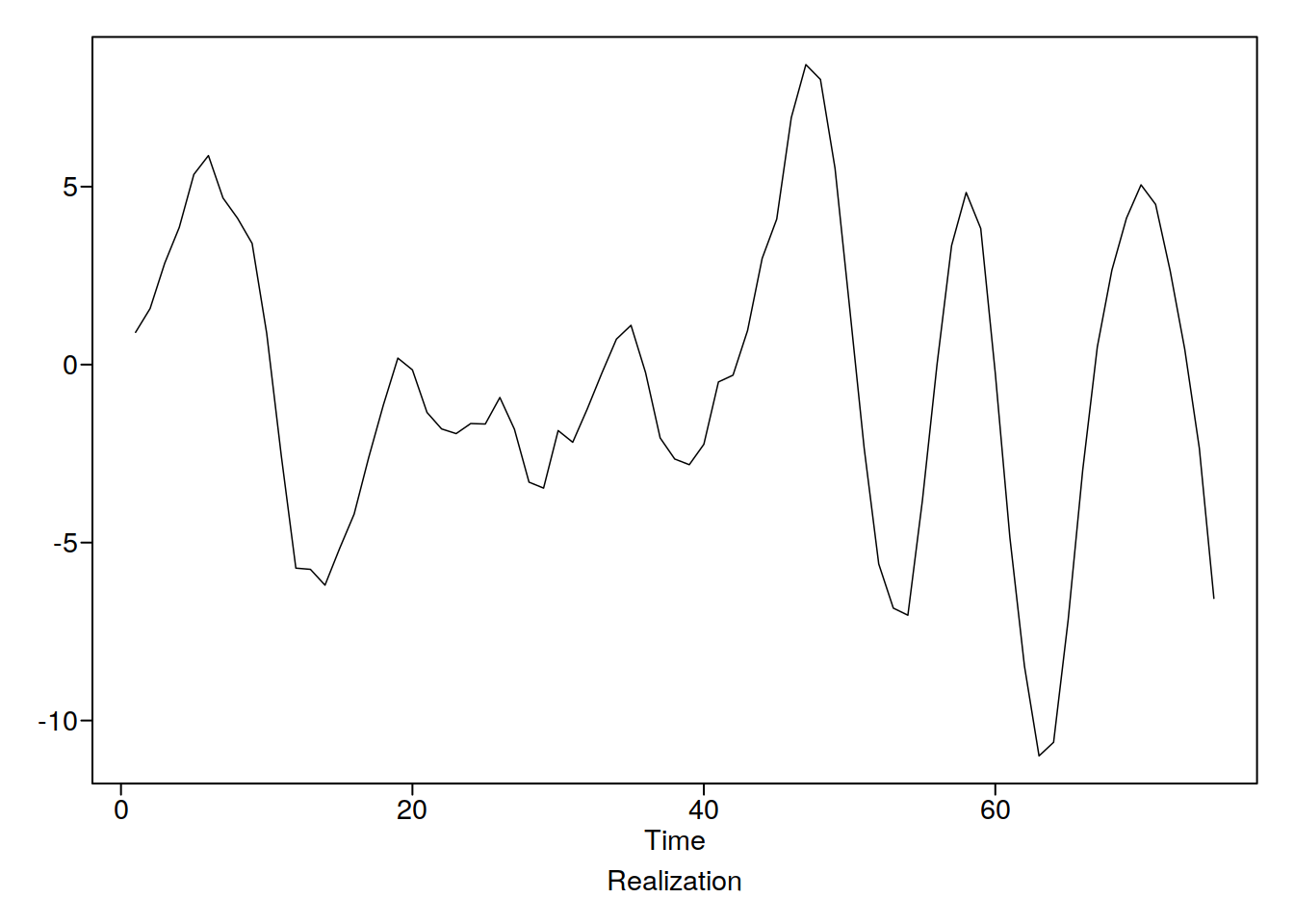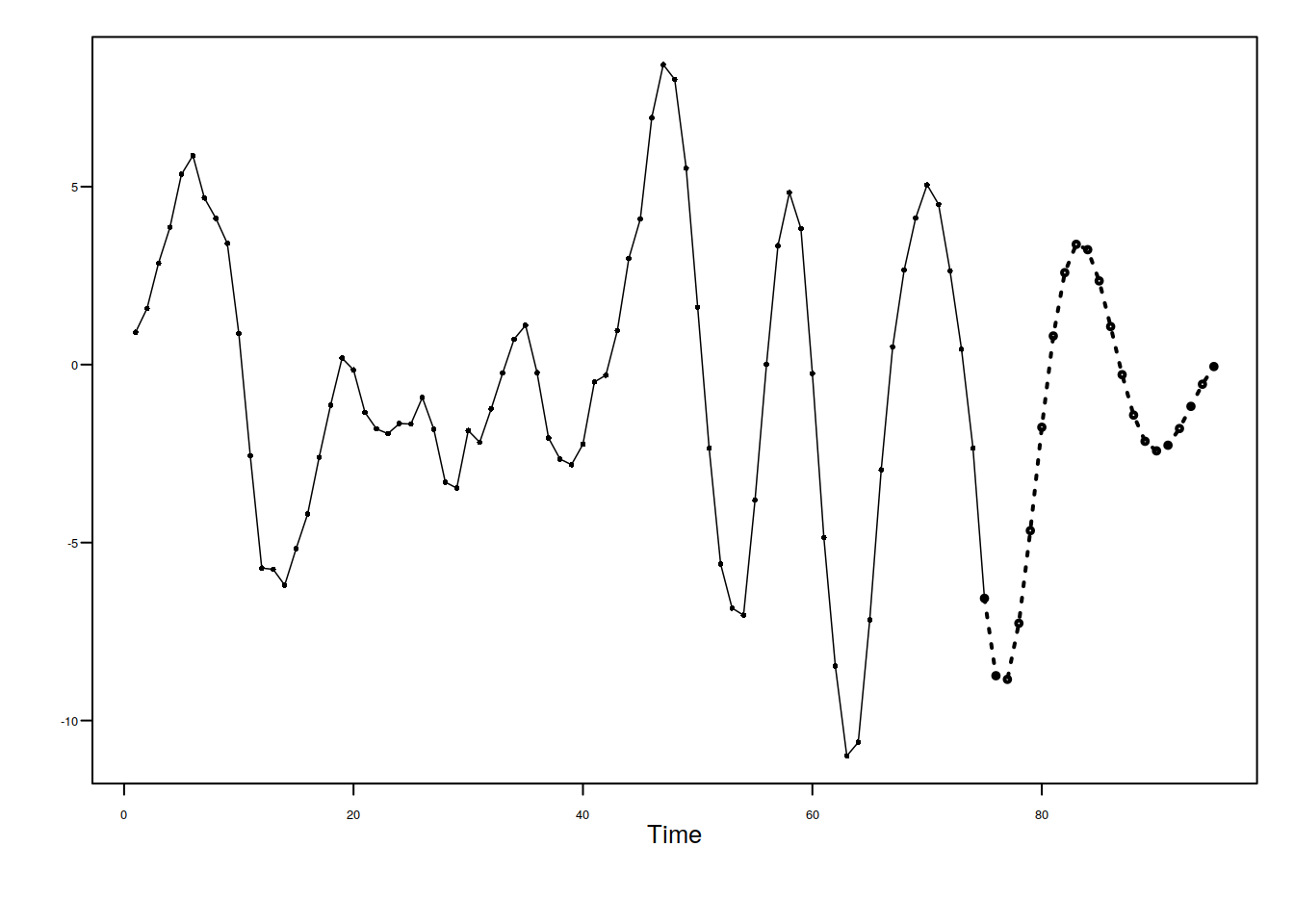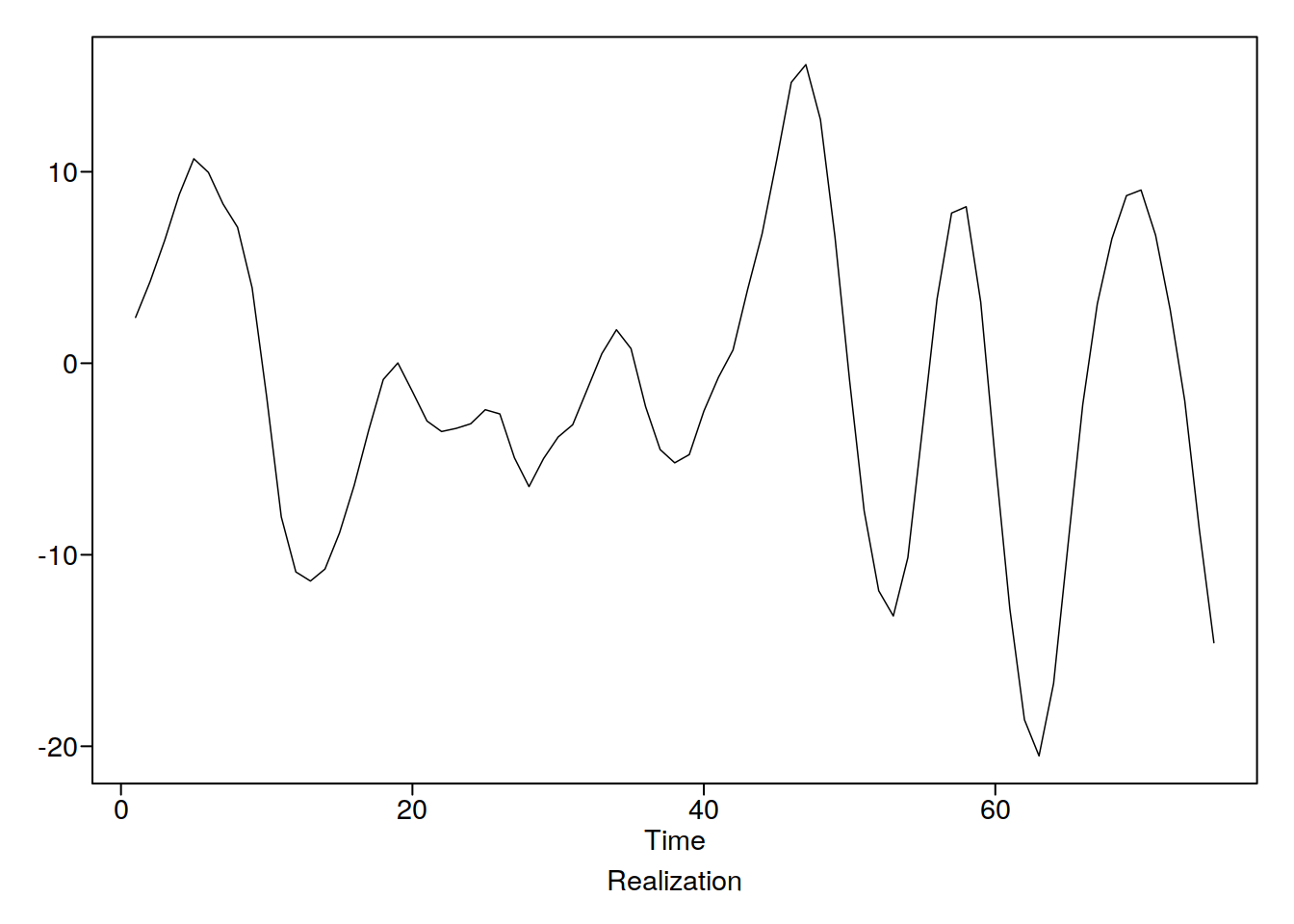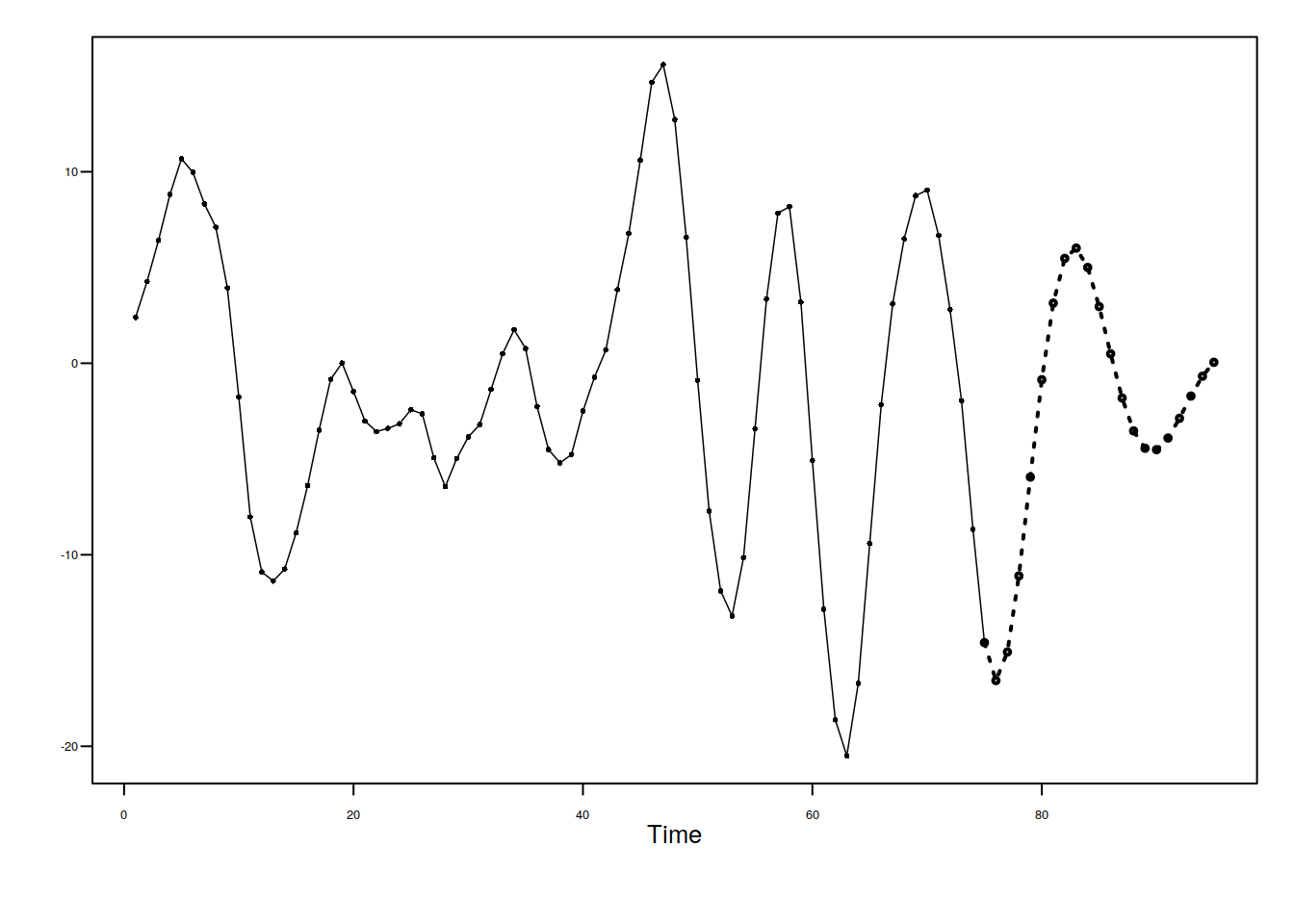# Unit 27 ARMA(p,q)

## 27.1 Some math

$\hat{X}_{t_0} (\ell) = \sum_{j = 1}^p \phi_j \hat{X}_{t_0}(\ell - j) - \sum_{j = \ell}^q \theta_j \hat{a_{t_0 + \ell - j}} + \bar{X} \left[ 1- \sum_{i = 1}^p \phi_i \right]$ Where the a hat is incredibly hard to calculate (we have to do back casting, which we can worry about after the test). Challeng: Write this function recursively (is it possible?????) in R. We are just going to use fore.arma.wge for now

ts7524 <- tswgen(75, sn = 24)
p <- c(1.6, -0.8)
thet <- (-0.9)
x1 <- ts7524$arma(phi = p)fore.arma.wge(x1, phi = p, n.ahead = 20, limits = F)x2 <- ts7524$arma(phi = p, theta = thet)fore.arma.wge(x2, phi = p, theta = thet, n.ahead = 20, limits = F)